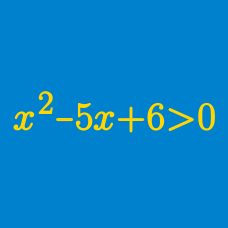Algebra

# Polynomial Inequalities: Level 4 Challenges

Juliet has attempted 213 problems on Brilliant and solved 210 of them correctly. Her friend Romeo has just joined Brilliant, and attempted 4 problems and solved 2 correctly. From now on, Juliet and Romeo will attempt all the same new problems. Find the minimum number of problems they must attempt such that it is possible that Romeo's ratio of correct solutions to attempted problems will be strictly greater than Juliet's.

As $t$ ranges over all real values, what is the minimum of $f(t) = (t^2-3)(t^2-5)(t^2-7)(t^2-9) + 100 ?$

$x^{2} + ax+a^{2} + 6a <0$

Find the sum of squares of all integral values of $a$ for which the inequality above is satisfied for all $1

Consider the function $f(x)=\dfrac{x^{2}+ax+b}{x^{2}+2x+3}$ where $a$ and $b$ are positive integers.

If the range of $f(x)$ is $[-5,4],$ find the value of $a^{2}+b^{2}-ab.$

Given that $a_1,a_2,\ldots,a_n,b_1,b_2,\ldots,b_n$ are non-negative real numbers that sum to 10, find the minimum possible value of $\left( \sum_{i=1}^{n} \sqrt{a_i^2+b_i^2}\right)^2.$

×